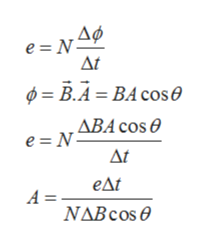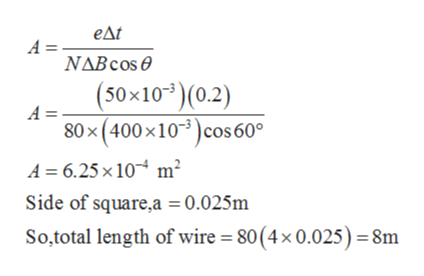# A coil formed by wrapping 80 turns of wire in the shape of asquare is positioned in a magnetic field so that the normal to the plane ofthe coil makes an angle of 60.0∘ with the direction of the field. When themagnetic field is increased uniformly from 100 mT to 500 mT in 0.20 s,the emf of magnitude 50.0 mV is induced in the coil. What is the totallength of the wire in the coil?

Question
9 views

A coil formed by wrapping 80 turns of wire in the shape of a
square is positioned in a magnetic field so that the normal to the plane of
the coil makes an angle of 60.0
∘ with the direction of the field. When the
magnetic field is increased uniformly from 100 mT to 500 mT in 0.20 s,
the emf of magnitude 50.0 mV is induced in the coil. What is the total
length of the wire in the coil?

check_circle

Step 1

Given,

Step 2

Emf in magnetic field is calculated as,help_outlineImage Transcriptionclosee N ΔΜ φ= Β.4-ΒA cos θ , ΔΒΑ cos θ e = N Δt eAt A = ΝΔBcos θ fullscreen
Step 3

By substituting the ...help_outlineImage Transcriptioncloseедt A = NABCOS (50x103)(0.2) А- 80x(400x103)cos 60° A 6.25 x 10 m2 Side of square,a = 0.025m So,total length of wire = 80(4x 0.025) = 8m fullscreen

### Want to see the full answer?

See Solution

#### Want to see this answer and more?

Solutions are written by subject experts who are available 24/7. Questions are typically answered within 1 hour.*

See Solution
*Response times may vary by subject and question.
Tagged in

### Electromagnetic Induction